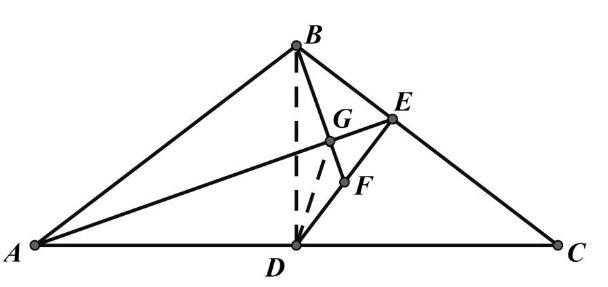【答案】

$\frac{B D}{D E}=\frac{D C}{C E}$
$\frac{B D}{D F}=2 \frac{B D}{D E}=2 \frac{D C}{C E}=\frac{A C}{C E}, \triangle B D F \sim \triangle A C E$
$\angle D B F=\angle C A E, \therefore A 、 D 、 G 、 B$ 四点共圆。
$\angle B G A=\angle B D A=90^{\circ} \quad, A E \perp B F$ 。